### Home > CCA2 > Chapter Ch11 > Lesson 11.1.2 > Problem11-26

11-26.
1.Use the values for ln 2 and ln 3 given in problem 11-25 to evaluate each of the following expressions. Do not use a calculator. Homework Help ✎

1. ln 6

2. ln 12

3. ln 16

4. ln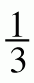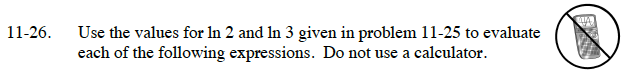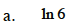ln6 = ln(2 · 3)

ln6 = ln2 + ln3

ln6 = (0.69315) + (1.0986)
ln6 = 1.7918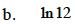See part (a).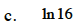See part (a).$\ln\frac{1}{3} = \ln\left(3^{-1}\right)$

$\ln\frac{1}{3} = -\ln3$